##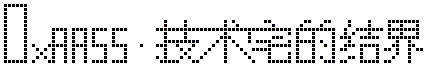帐号 自动登录 找回密码 密码 立即注册→加入我们
 搜索 热搜: 下载 VB C 实现 编写

# 【VFB】FreeBASIC语言入门（VFB教程2-1）

51主题 642积分

UID
3260

7

12 点

502 个

1 次

0 份

22 小时

2017-12-26发表于 2018-2-26 20:53:28 | 显示全部楼层 |阅读模式

### 欢迎访问技术宅的结界，请注册或者登录吧。

x

[Visual Basic] 纯文本查看 复制代码
Dim

Print

For...Next

If...Then

Do...Loop

[Visual Basic] 纯文本查看 复制代码
Print "你好，世界！"

[Visual Basic] 纯文本查看 复制代码
Dim text As String
text = "你好，世界！"
Print text

[Visual Basic] 纯文本查看 复制代码
Dim a As String, b As String, text As String
a = "你好"
b = "世界"
text = a + " " + b + "!"
Print text

[Visual Basic] 纯文本查看 复制代码
Dim a As Integer, b As Integer, c As Integer

a = 5
b = 7
c = a + b

Print "a = "; a
Print "a = "; b
Print "a + b = "; c

[Visual Basic] 纯文本查看 复制代码
Dim total As Integer
Dim number As Integer
total = 0
For number = 1 To 100
total = total + number
Next
Print "The sum of number from 1 to 100 is "; total

[Visual Basic] 纯文本查看 复制代码
Dim number As Integer
number=1
If number < 0 Then
Print "negative"
ElseIf number > 0 Then
Print "positive"
Else
Print "zero"
End If

[Visual Basic] 纯文本查看 复制代码
Dim total As Single, count As Single, number As Single
Dim text As String

Print "This program will calculate the sum and average for a"
Print "list of numbers.  Enter an empty value to end."
Print

Do
Input "Enter a number : ", text
If text = "" Then
Exit Do
End If

count = count + 1
total = total + Val(text)

Loop

Print
Print "You entered "; count; " numbers"
Print "The sum is "; total
If count <> 0 Then
Print "The average is "; total / count
End If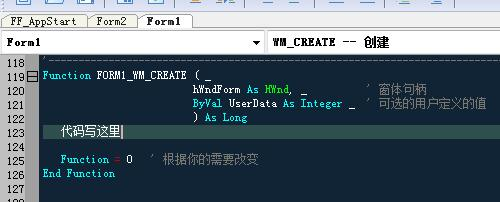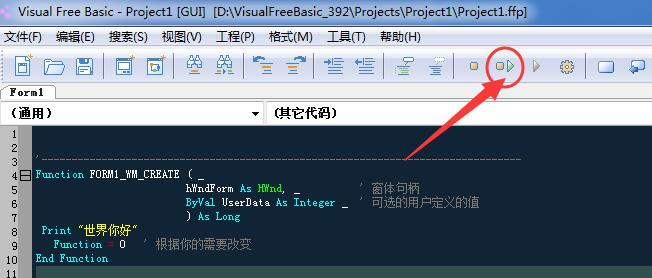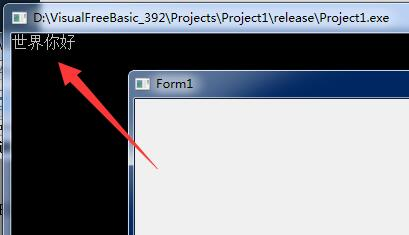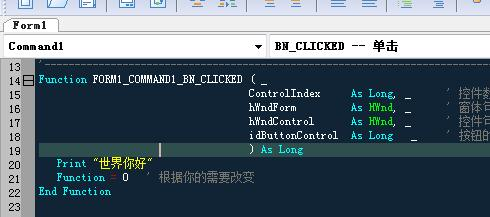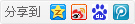本版积分规则 回帖并转播 回帖后跳转到最后一页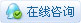|申请友链||Archiver|手机版|小黑屋|技术宅的结界 ( 滇ICP备16008837号 )|网站地图

GMT+8, 2020-6-4 22:47 , Processed in 0.092442 second(s), 33 queries , Gzip On.